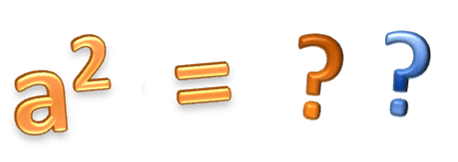Problems
235 - Simply Square

Simply Square

Time Limit: 1 sec

The Problem

Squaring is the simplest thing of math. It’s a simple multiplication. A number, a and some instructions will be given and you have to solve the problem by following those instructions.Step 1: Squaring the given number such as:

If a = 32

322

= 1024

1 + 0 + 2 + 4

= 7

72

= 49

Step 4: Subtract step 3’s answer from step 1

1024 – 49

= 975

9752

= 950625

Step 6: Print the value of 1’s digit

5

The Input

Input file contains a single integer a (32<=a<=99).

The Output

Output will show the 1’s digit of the answer in a separate line.

Sample Input

32

Sample Output

5

Problem Setter: Samia Safa Ahmed